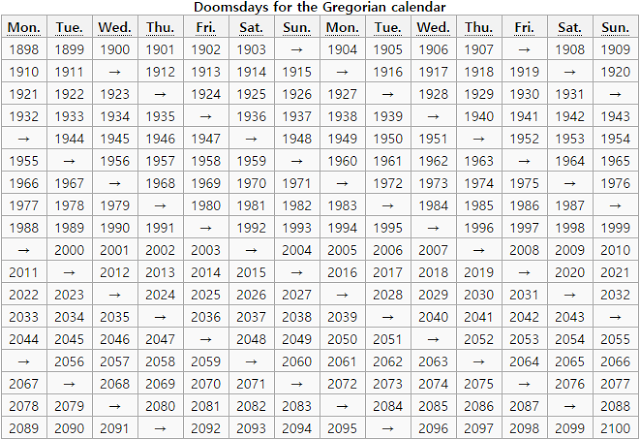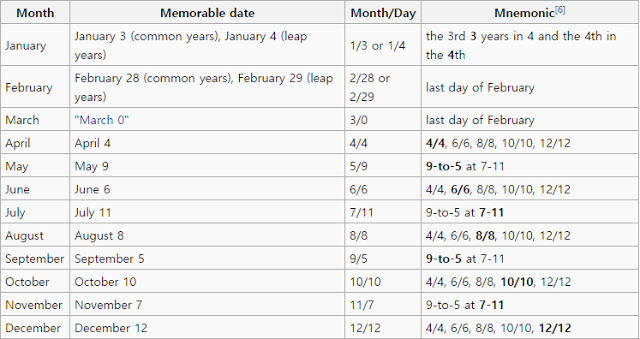# Doomsday algorithm in C language

## What is doomsday?

The doomsday rule or doomsday algorithm is a way of calculating the day of the week of a given date. It provides a perpetual calendar because the Gregorian calendar moves in cycles of 400 years.

## Applying doomsday algorithm

1. Determine the `"anchor day"` for the century.
2. Use the anchor day for the century to calculate the doomsday for the year.
3. Choose the closest date out of the ones that always fall on the doomsday. (e.g. 4/4, 6/6, 8/8), and count the number of days (modulo 7, %7 in C) between that date and the date in question to arrive at the date of the week.## How to calculate doomsday of the year

Here are the list of doomsday standard for years.
1800~1899 is Friday
1900~1999 is Wednesday
2000~2099 is Tuesday
2100~2199 is Sunday
Then it loops

Using the data above, we can calculate a doomsday of the year we choose.
For example I will choose 2016 03 24 Formatted as YYYYMMDD.

1. Divide last two digits of Year with 12 - YYYY -> YYYY / 12 -> a…b
`2016-> 16/12 = 1 ... 4 as remainder ( a is 1 and b is 4 )`
2. Divide the remainder with 4 -> `b/4`
`4/4 = 1 (c is 1)`
3. Anchor day can be calculated with list above with YYYY and add a+b+c on the anchor day.
`2016 will be between 2000~2099, Tuesday.`
`a+b+c = 1 + 4 + 1 = 6`
`Now add result from 2 on Tuesday. Which will be Tuesday + 6 = Monday.`
`2016's doomsday is Monday`

## Calculate a given date

Now we know 2016’s doomsday is on Monday. Then we can simply calculate the difference in date between doomsday and the day we want to know, to calculate the day.

Using the example above, 2016 03 24, we know 2016’s doomsday is on Monday and I will use February 29th for my doomsday since it’s a leap year.

Days until February 29th are 60 days and days until 24th of March is 84 days. Now we can calculate the day with the function I wrote on the program.

`dayOfWeek = ((doomsday + DAYS_PER_WEEK) - (DAYS_PER_WEEK + ((daysToEndOfFeb - daysToInputDay) % DAYS_PER_WEEK))) % DAYS_PER_WEEK;`

This will give me a day of week with leap year case.

`dayOfWeek = ((4 + 7) - (7 + ((60 - 84) % 7))) % 7;`
`= ((11) - (7 + (-3))) % 7;`
`= 7 % 7`
`= 0`
`= Thursday`